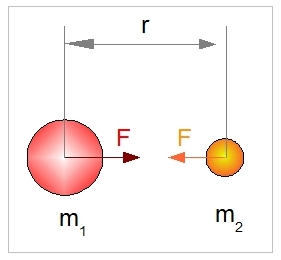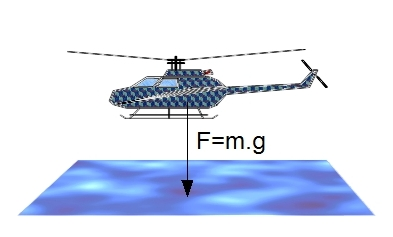# Gravitation & Weight

## Gravitation

Gravitation is the force that describes the gravitational attraction between two bodies with mass. The gravitational attraction force is defined by Newton's universal law of gravitation (classical physics).

F = G. (m1 . m2 ) / r2

where:
G: Gravitational constant with the value of 6.67 × 10−11 N m2 kg-2
M1: The mass of body 1 in kg
M2: The mass of body 2 in kgWe will use this formula to calculate the force between an object and the earth. We can make the following observations: the distance r is a practical constant when considering objects that are close to the surface of the earth. By substituting G, r and the mass of the earth with their values, we get:

F = 9.8 m

Compare this to the formula F = m.a, and it follows that for objects in the vicinity of the earth's surface, the acceleration 'a' equals 9.8 m/s2.
This value is called the earth's gravitational acceleration g.

F = g . m

where:
F : Force in N
g : Gravitational acceleration in m/s2, with g = 9.8
m : Mass in kg

## Weight

When an object is attracted with a force F = g.m, the weight of the object resting on a solid surface is defined as the reaction force against the surface.

## Explanation

The force that an object encounters when near to the earth's surface can be calculated from the universal law of gravitational attraction. It appears that a body with mass, m, encounters an acceleration force, g, of 9.8 m/s2. This results in the earth's gravitational force, F = g.m. The assumptions we make is that the object's mass is much less than the mass of the earth. The same must apply to the diameters. The earth's diameter must be much bigger than the object’s diameter. These assumptions hold true at the earth’s surface, but when looking, for example, at the gravitational attraction forces between bodies in space and the earth (e.g. the moon and sun) these assumptions do not apply.

In aviation, these assumptions generally hold true, with the only factor of influence being the distance to the earth. Let’s look at the influence of the distance to earth with an example.

An airplane is flying at a height of 10 Km (32,808 feet). By what amount is the plane attracted to the earth compared to a grounded airplane?
Answer: The difference in attraction force at a height of 10 km can be found by:
100% . (1/r2 - 1/(r + 10)2) / (1/r2)
Diameter earth:  = 12,756 Km, r = 6378 Km.

1/r2=1/63782 = 2,4582778622933706834238618738901e-8
1/(r + 10)2 = 1/(6378+10)2 = 2,4505873371682737945168794495314e-8

(2,4583 - 2,4506) / 2,4583  = 0,0031 -> 0,31%

Conclusion, at a height of 10 km (32,808 feet) an aircraft will experience 0.31% less gravitational attraction force. In most cases, this is of no concern.

## Examples

A helicopter with a mass of 500 Kg is flying in a hover at a height of 2 m (6.6 feet).  How great is the gravitational force working on the helicopter which must be overcome by the vertical thrust of the rotor system?
F = g.m = 9.8 . 500 = 4900 N.A helicopter is diving down, and for a brief moment reaches an acceleration of -9.8 m/s2 (the acceleration is directed towards the earth's surface). What is the weight the pilot will experience sitting in his seat? The pilot has a mass of 95kg.
Weight is a reaction force. There are two acceleration forces acting upon the pilot. The first is due to the gravitational acceleration, g. The second is due to the acceleration of the helicopter (relative to the earth's surface):

F1 = m . g
F2 = m . a

g = 9.8
a = -9.8

Ftotal = F1 + F2 = m . (9.8 - 9.8) = 0 N
The pilot experiences weightlessness.

Now, the helicopter starts decelerating in a vertical direction at a rate of 5 m/s2. What is the weight of the pilot?
Ftotal = 95  (9.8 + 5) = 1406N. Compared to the norm (only gravitational pull), the weight has increased by (9.8 + 5) / 9.8, or 51%. Of course, the mass remains at 95kg. Note that this increase in force working on the pilot leads to a reaction force at the seat, holding him in place.

# Do you want to comment this topic?

1: (Book) Cyclic and Collective2: (Book) Principles of Helicopter Flight4: Logitech Extreme 3D Pro Joystick5: Saitek Pro Flight Rudder Pedals